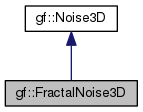Gamedev Framework (gf)  0.2.0 A C++11 framework for 2D games
gf::FractalNoise3D Class Reference

Fractal 3D noise. More...

#include <gf/Noises.h>

Inheritance diagram for gf::FractalNoise3D:[legend]

## Public Member Functions

FractalNoise3D (Noise3D &noise, double scale, std::size_t octaves=8, double lacunarity=2.0, double persistence=0.5, double dimension=1.0)
Constructor. More...

virtual double getValue (double x, double y, double z) override
Take a 3D noise value. More...Public Member Functions inherited from gf::Noise3D
virtual ~Noise3D ()
Virtual destructor. More...

double operator() (double x, double y, double z)
Take a 3D noise value. More...

## Detailed Description

Fractal 3D noise.

Fractal noise is based of fractional Brownian motion (fBm). It consists in adding several octaves of a basic noise at different amplitudes.

## Constructor & Destructor Documentation

 gf::FractalNoise3D::FractalNoise3D ( Noise3D & noise, double scale, std::size_t octaves = 8, double lacunarity = 2.0, double persistence = 0.5, double dimension = 1.0 )

Constructor.

Parameters
 noise The basic noise function scale The scale factor octaves The number of octaves lacunarity The factor applied to frequency persistence The factor applied to amplitude dimension The contrast between the layers

## Member Function Documentation

 virtual double gf::FractalNoise3D::getValue ( double x, double y, double z )
overridevirtual

Take a 3D noise value.

Parameters
 x The x coordinate of the noise value y The y coordinate of the noise value z The z coordinate of the noise value
Returns
The noise value

Implements gf::Noise3D.